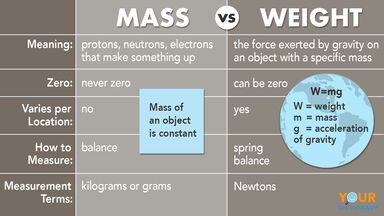# Mass vs. Weight: Simple Breakdown of the Differences

Get a clear understanding of the difference between mass vs. weight. See how both are measured and why weight is different than mass. Learn a mnemonic device to help you remember the difference between weight and mass.mass vs weight comparison examples

## The Difference Between Mass vs. Weight

It might be challenging to understand, but mass and weight are not the same thing. On Earth, you use mass and weight interchangeably because they are equal. The key to that phrase is “on Earth,” if you weren’t on Earth, your mass and weight would not be equal. Why? To answer that question, look at their definitions.

• Mass is the weight of the stuff making up an object measured in kilograms.
• Weight is the gravitational force interacting with Earth. You measure weight in Newtons.

On Earth, the mass of an object and the weight of an item are proportional. So, your weight and mass are both 150 pounds or about 68 kilograms. This is also about 11 stone.

### Difference Between Mass and Weight

Before getting into the details of the difference between mass and weight, it can help to break down the differences into an easy-to-follow chart.

 Term: mass weight Meaning: protons, neutrons, electrons that make something up the force exerted by gravity on an object with a specific mass Zero: never zero can be zero Varies According to Location: no yes How to Measure: balance spring balance Measurement terms: kilograms or grams Newtons

## What Is Mass?

When it comes to mass, it’s the measure of all the different protons, electrons and neutrons that make up something. This something could be a chair, atom or person. When it comes to your mass, it’s constant. Your mass on Earth is the same as it is on the sun or Jupiter. The mass of an object is constant. Mass is also a scalar quantity, so it has magnitude.

### How Is Mass Measured?

Since mass is constant, there is a constant way to measure it. Mass is measured in grams or kilograms. For example, gold has a mass of 19.3 grams. Scientists measure the mass of an object using a balance. A balance is a perfect way to measure mass because a balance isn’t affected by gravity. It gives you the accurate mass of an object.

## What Is Weight?

Now that you know what mass is, it’s time to look at weight. Weight (W) is found by taking mass (m) and multiplying it by the acceleration of gravity (g), which can be written with the equation W = mg. The weight of an object can vary based on gravity; therefore, weight is not constant.

For example, your weight on the moon is only 16.5% of that of Earth. So, if you weigh 220 pounds on Earth, then you weigh 36 pounds on the moon. Who needs to diet? Everyone just needs to live on the moon. Additionally, weight has both magnitude and direction; it’s a vector quantity.

### How Is Weight Measured?

While your scale might tell you your weight is measured in kilograms, pounds or stone, weight is measured in Newtons in the scientific world. And since you need to measure the gravity exerted on the object, you use a spring balance.

## How to Remember Mass vs. Weight

Since mass and weight are the same in some cases, it can be easy to get them confused on Earth. Therefore, you might want to use a memory trick to help you remember. Try this phrase when trying to remember mass vs. weight.

My mass is always the same, while my weight depends on gravity.

## Mass vs. Weight: A Weighted Debate

Weight and mass can be easy to confuse on Earth because they are essentially the same measurement. However, when you get to space, it’s a whole new ballgame. Now that you have the weight vs. mass problem down to a science, you can move on to bigger and better things. You might do this by taking a gander at weather vs. whether. Do you know which one to use? From there, keep the science exploration going by discovering the difference between silicon and silicone.